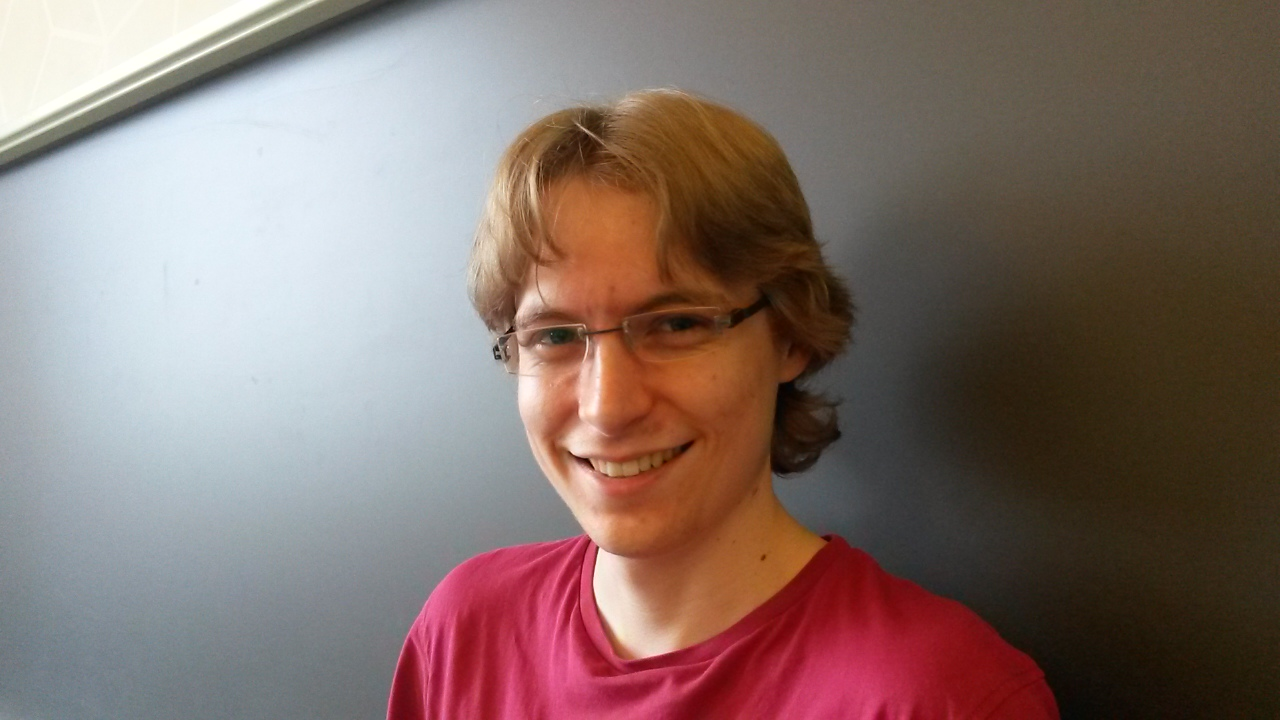Johannes Schmitt
I am a Postdoc in Bonn working with Daniel Huybrechts. Previously, I was a PhD student of Rahul Pandharipande at ETH Zürich. My CV can be found here.
ContactEmail schmitt@math.uni-bonn.de Phone +49 228 73 62258 Address Endenicher Allee 6053115 BonnGermany Office 3.002

Teaching

V5A4 - Selected Topics in Algebraic Geometry - The moduli space of curves (Summer term 2020)
Research Interests

Algebraic geometry, Moduli spaces of curves, Geometric Invariant Theory, Algebraic stacks, Tautological cycles, Hurwitz spaces, Chow groups
Papers/Preprints

Algebraic geometry
• Tevelev degrees and Hurwitz moduli spaces (joint with A. Cela, R. Pandharipande)
Preprint (2021) - preprint
• The Hodge bundle, the universal 0-section, and the log Chow ring of the moduli space of curves (joint with S. Molcho, R. Pandharipande)
Preprint (2021) - preprint
• Chow rings of stacks of prestable curves (joint with Y. Bae)
Preprint (2020) - preprint
• Pixton's formula and Abel-Jacobi theory on the Picard stack (joint with Y. Bae, D. Holmes, R. Pandharipande, R. Schwarz)
Preprint (2020) - preprint
• admcycles -- a Sage package for calculations in the tautological ring of the moduli space of stable curves (joint with V. Delecroix, J. van Zelm)
Preprint (2020) - preprint
• Infinitesimal structure of the pluricanonical double ramification locus (joint with D. Holmes)
Preprint (2019) - preprint
• Zero cycles on moduli spaces of curves (joint with R. Pandharipande)
Épijournal de Géométrie Algébrique, 4. (2020) - preprint, journal version
• Intersections of loci of admissible covers with tautological classes (joint with J. van Zelm)
Selecta Mathematica, 26. (2020) - preprint, journal version
• Multiplicativity of the double ramification cycle (joint with D. Holmes, A. Pixton)
Documenta Mathematica, 24. (2019) - preprint, journal version
• A correspondence of good G-sets under partial geometric quotients
Beiträge zur Algebra und Geometrie, 59.2 (2018) - preprint, journal version
• Dimension theory of the moduli space of twisted k-differentials
Documenta Mathematica, 23. (2018) - preprint, journal version
• A compactification of the moduli space of self-maps of $$\mathbb{CP}^1$$ using stable maps
Conformal Geometry and Dynamics, 21. (2017) - preprint, journal version
Other subjects
• Detecting and Counting Small Subgraphs, and Evaluating a Parameterized Tutte Polynomial: Lower Bounds via Toroidal Grids and Cayley Graph Expanders (joint with M. Roth, P. Wellnitz)
Preprint (2020) - preprint
• Counting Small Induced Subgraphs Satisfying Monotone Properties (joint with M. Roth, P. Wellnitz)
FOCS conference 2020 - preprint
• Counting Induced Subgraphs: An Algebraic Approach to #W-hardness (joint with J. Dörfler, M. Roth, P. Wellnitz)
MFCS conference 2019 - preprint, conference version
• Counting Induced Subgraphs: A Topological Approach to #W-hardness (joint with M. Roth)
IPEC conference 2018 (Best Paper Award and Best Student Paper Award), Algorithmica, 82. (2020) - preprint, journal version
• Cowen-Douglas operators and dominating sets (joint with J. Eschmeier)
Journal of Operator Theory, 1. (2014) - preprint, journal version
Lecture notes

The moduli space of curves (Summer 2020)

admcycles
A Sage package for calculations in the tautological ring of $$\overline{\mathcal{M}}_{g,n}$$ and computation of cycles of admissible covers (joint with V. Delecroix, J. van Zelm)

Organization

Hausdorff school “Perverse Sheaves in Enumerative Geometry” (February 10-14, 2020).
Online working seminar “Smooth compactifications and bChow intersections via blowups/subdivisions” (Summer 2020).

Some Links & Files
1. Operators of Cowen-Douglas class, Bachelor thesis, 2012
2. The Toric Variety $$\text{Bl}_{n+1} \mathbb{P}^n$$ and its Associated Secondary Fan, Master thesis, 2014
3. A poster concerning the moduli spaces of self-maps of $$\mathbb{CP}^1$$, presented at the AMS Summer Institute 2015 in Salt Lake City
4. A poster about spaces of twisted $$k$$-differentials, presented at the GAeL-conference 2017 in Bath
5. Slides from a beamer talk about a conjectural relation of moduli spaces of twisted $$k$$-differentials and Pixton's class
6. Slides from my PhD defense talk
7. A talk about the relation between strata of k-differentials and double ramification cycles
8. Another talk about the relation between strata of k-differentials and double ramification cycles
9. An (interactive) talk about the software package admcycles, given in Zurich
10. Another (interactive) talk about the software package admcycles, given in Leiden
11. A talk about a paper by Pandharipande-Yin about relations in the Chow group of the moduli of K3 surfaces

LaTeX formulas on this site powered by MathJax